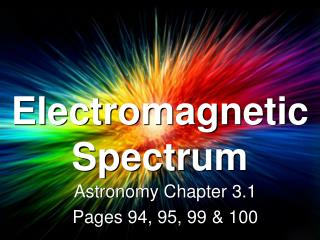DownloadDownload PresentationElectromagnetic Spectrum

# Electromagnetic Spectrum

Télécharger la présentation## Electromagnetic Spectrum

- - - - - - - - - - - - - - - - - - - - - - - - - - - E N D - - - - - - - - - - - - - - - - - - - - - - - - - - -
##### Presentation Transcript

1. Electromagnetic Spectrum Astronomy Chapter 3.1 Pages 94, 95, 99 & 100

2. Electromagnetic Radiation • Travel in the form of waves • These waves can travel through empty space.

3. Electromagnetic Spectrum • ER waves travel at the speed of light • 300,000km per second • Wavelength, the length from one wave to another, determines the type of radiation

4. D. Gamma Rays B. Visible C. Ultraviolet A. Radio Waves Which of the following has the longest wavelength?

5. The Sun • Emits electromagnetic radiation of all types.

6. Electromagnetic Spectrum • The various types of ER waves arranged on a continuum

7. What is Visible Light? • A form of electromagnetic radiation (EMR) that you can see • A form of energy that travels in waves like: • Radio waves • X-rays • Visible light

8. Spectrum • Visible Light • Made of various colors, each with a different wavelength • These are the colors seen in a rainbow.

9. A. Red F. Violet C. Yellow D. Green B. Orange E. Blue Which color has the shortest wavelength?

10. Spectrum (Prisms) • When white light is passed through a prism, the light is bent into the visible spectrum.

11. Spectrum R – O – Y – G – B – I – V • (Red, Orange, Yellow, Green, Blue, Indigo, Violet)

12. Spectroscope • Uses a prism to split the light gathered by a telescope into a spectrum.

13. Three types of Spectra • Three different types • Continuous Spectrum • Emission Spectrum • Absorption Spectrum

14. Continuous Spectrum • Unbroken band of colors • Shows that the source is emitting all visible wavelengths.

15. Emissions Spectrum • A series of unevenly spaced lines of different colors and brightness. • The bright lines show that the source is emitting light of certain wavelengths.

16. Emissions Spectrum • Produced by glowing gases • Every element has a unique emission spectrum • Allows scientists to determine the elements in objects.

17. Absorption Spectrum • A continuous spectrum crossed by dark lines • Lines form when light from a glowing object passes through a cooler gas. • The gas absorbs the wavelengths that are missing.

18. Absorption Spectrum • The colors absorbed are the same that the gas would emit • Absorption Spectrum for Hydrogen • Emission Spectrum for Hydrogen

19. D. Absorption Spectrum B. Emission Spectrum C. Continuous Spectrum A. Visible Which spectrum would you expect to see produced by glowing gasses?

20. D. Absorption Spectrum B. Emission Spectrum C. Continuous Spectrum A. Visible Which spectrum would you expect to see if you were studying the gasses on another planet?

21. Absorption Spectrum • How do these spectrum allow astronomers to figure out what elements make up the atmospheres of stars and planets?

22. Chemical Composition • Chemical elements in a star’s atmosphere absorb light from the star. • Each element absorbs a different wavelength. Each absorbed wavelength is shown as a dark line on a spectrum.

23. Chemical Composition • It acts like a finger print each element has a unique set of lines. • By comparing the star’s spectrum to the known spectrums of different elements, astronomers can determine the elements present.

24. Temperatures • Most stars have a chemical composition similar to the sun. 73% H, 25%He and 2% other elements. • Stars at different temps produce different line spectrums. • If the star is at about 10,000˚C it produces a very strong spectral lines for H. • If the hydrogen line is not strong, it means the star is cooler than 10,000˚C

25. Absorption Spectrums Star A Hydrogen Helium Sodium Calcium

26. Absorption Spectrums Star B Hydrogen Helium Sodium Calcium

27. Absorption Spectrums Star C Hydrogen Helium Sodium Calcium

28. Constellations • Patterns of stars in the sky • ORION- The Hunter